# We Helped With This MATLAB Programming Assignment: Have A Similar One?

SOLVEDCategory Programming MATLAB Undergraduate Solved Pay For Matlab Homework

## Assignment Description

Need to be done in MATLAB Question (1):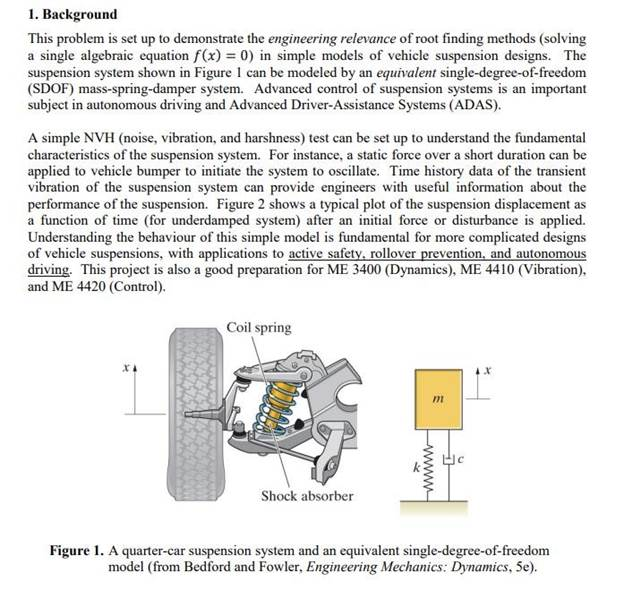For this question 1 Part (a) code has been given and not sure if it’s done and need part(b) . So here is the code for part (a)

%% Script file project1.m

%% Definition of system parameters: global m c k global x0 v0 m = 1e3;     k = 81e3;    % c          x0 = 1;

v0 = 0;

%% Part (a)

% Plot the time response and total mechanical energy of the SDOF system

% for the different cases of damping being considered.

% Note: Project asks for only the cases of c = [3 18 25]*1e3

% c = 3e3 N.s/m   % under-damped case (zeta = 0.167)

% c = 18e3 N.s/m  % critically damped case (zeta = 1) % c = 25e3 N.s/m  % overdamped case (zeta = 1.389) c_values = [3 18 25]*1e3;

% Plot of the time response figure

for j = 1:length(c_values)     c = c_values(j);

% plot the free response normalized with respect to x0     fplot(@(t) frsp_sdof(t)/x0, [0,2]), hold on end hold off

% Set plot parameters % set(gca,'FontSize',12) ax = gca; ax.YLim = [-0.8,1]; ax.XTick = (0:0.2:2); ax.YTick = (-0.8:0.2:1); ax.FontSize = 11; ax.LineWidth = 1;

grid, xlabel('t [sec]'), ylabel('x/x_0 (normalized response)') title({'Time history of the free response of a suspension system', ...

'modeled by a single-degree-of-freedom system'})

LEG = legend('c = 3e3 N-s/m','c = 18e3 N-s/m','c = 25e3 N-s/m'); set(LEG, 'FontSize', 12)

% Plot of the mechanical energy versus time figure

E0 = 0.5*k*x0^2 + 0.5*m*v0^2;  % calculate the initial mechanical energy in

[J]

for j = 1:length(c_values)     c = c_values(j);

vel = derivative(@(t) frsp_sdof(t));  % define the velocity function

% Define the energy function (E), which is the sum of the potential     % and kinectic energies.  Normalize E w.r.t. the initial energy E0.     E=@(t) (0.5*k*frsp_sdof(t).^2 + 0.5*m*vel(t).^2)/E0;     fplot(E, [0,2]), hold on end hold off

% Set plot parameters ax = gca; ax.YLim = [0,1]; ax.XTick = (0:0.2:2); ax.YTick = (0:0.1:1); ax.FontSize = 11; ax.LineWidth = 1; grid, xlabel('t [sec]'), ylabel('E/E_0 (normalized energy)') title({'Time history of the total mechanical energy', ...

'of a suspension system modeled by a SDOF system'}) LEG = legend('c = 3e3 N-s/m','c = 18e3 N-s/m','c = 25e3 N-s/m'); set(LEG, 'FontSize', 12)

%% Part (b)

function x = frsp_sdof(t)

% Free response function of a SDOF system global m c k global x0 v0

wn = sqrt(k/m);         % natural frequency ("omega_n") zeta = c/2/sqrt(k*m);   % damping ratio wd = wn*sqrt(1-zeta^2); % damped natural frequency ("omega_d")

if zeta == 1;     x = x0*exp(-wn*t) + (v0+wn*x0)*t.*exp(-wn*t);  % critically damped case else

x = exp(-zeta*wn*t).*(x0*cos(wd*t)+(v0+zeta*wn*x0)/wd*sin(wd*t)); end

function df = derivative(f) sym_f = sym(f);

df = matlabFunction(simplify(diff(sym_f)));  % simplify command is optional end

Question (2)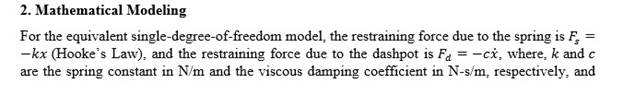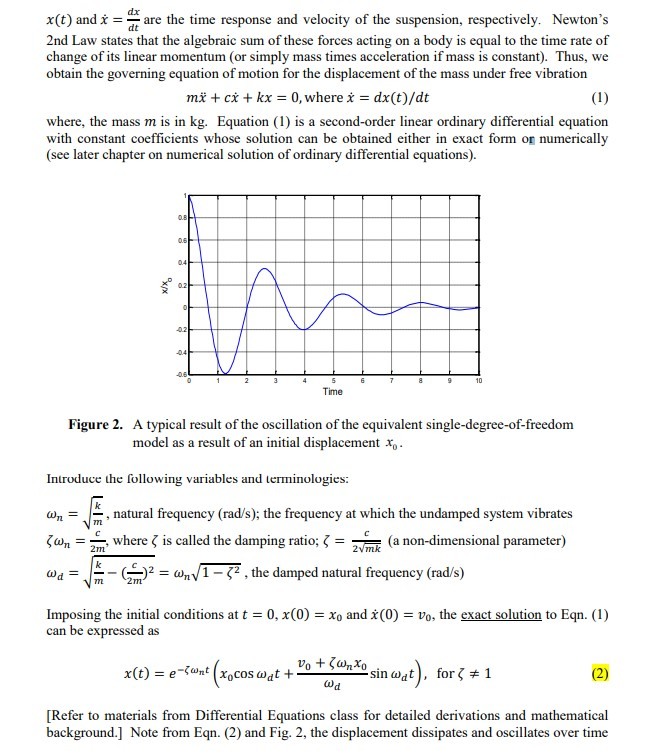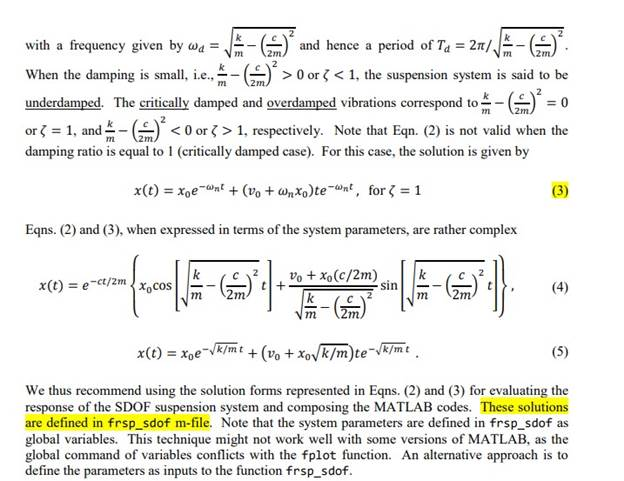Question (3):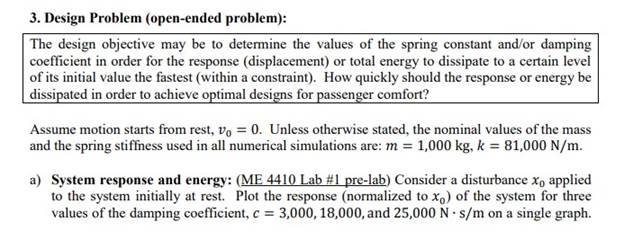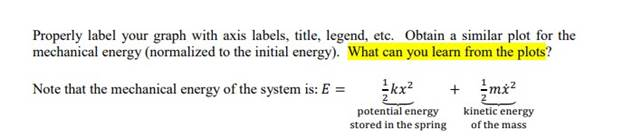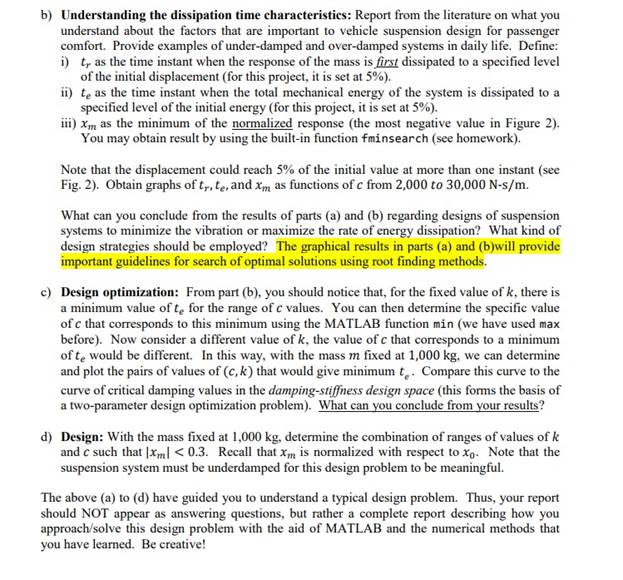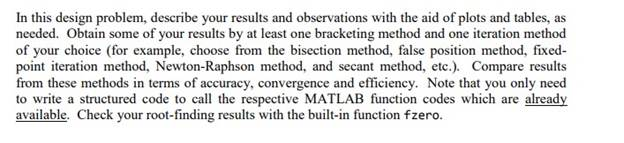Is it free to get my assignment evaluated?

Yes. No hidden fees. You pay for the solution only, and all the explanations about how to run it are included in the price. It takes up to 24 hours to get a quote from an expert. In some cases, we can help you faster if an expert is available, but you should always order in advance to avoid the risks. You can place a new order here.

How much does it cost?

The cost depends on many factors: how far away the deadline is, how hard/big the task is, if it is code only or a report, etc. We try to give rough estimates here, but it is just for orientation (in USD):

 Regular homework \$20 - \$150 Advanced homework \$100 - \$300 Group project or a report \$200 - \$500 Mid-term or final project \$200 - \$800 Live exam help \$100 - \$300 Full thesis \$1000 - \$3000

How do I pay?

Credit card or PayPal. You don't need to create/have a Payal account in order to pay by a credit card. Paypal offers you "buyer's protection" in case of any issues.

Why do I need to pay in advance?

We have no way to request money after we send you the solution. PayPal works as a middleman, which protects you in case of any disputes, so you should feel safe paying using PayPal.

Do you do essays?

No, unless it is a data analysis essay or report. This is because essays are very personal and it is easy to see when they are written by another person. This is not the case with math and programming.

Why there are no discounts?

It is because we don't want to lie - in such services no discount can be set in advance because we set the price knowing that there is a discount. For example, if we wanted to ask for \$100, we could tell that the price is \$200 and because you are special, we can do a 50% discount. It is the way all scam websites operate. We set honest prices instead, so there is no need for fake discounts.

Do you do live tutoring?

No, it is simply not how we operate. How often do you meet a great programmer who is also a great speaker? Rarely. It is why we encourage our experts to write down explanations instead of having a live call. It is often enough to get you started - analyzing and running the solutions is a big part of learning.

What happens if I am not satisfied with the solution?

Another expert will review the task, and if your claim is reasonable - we refund the payment and often block the freelancer from our platform. Because we are so harsh with our experts - the ones working with us are very trustworthy to deliver high-quality assignment solutions on time.

## Popular Solved Assignments Like This

Customer Feedback

"Thanks for explanations after the assignment was already completed... Emily is such a nice tutor! "

Order #13073